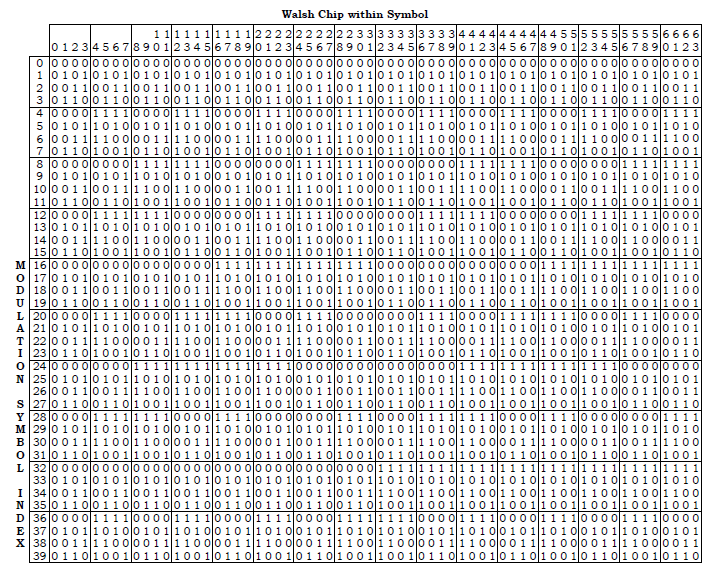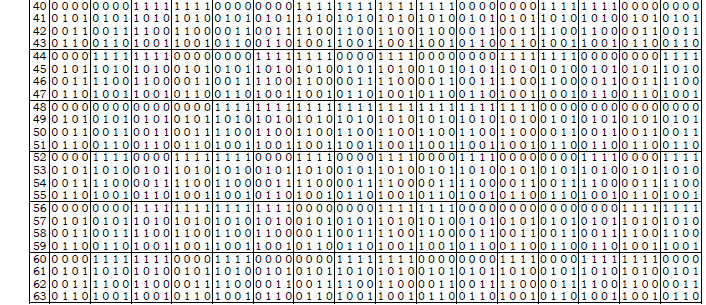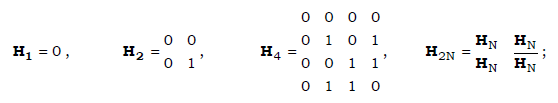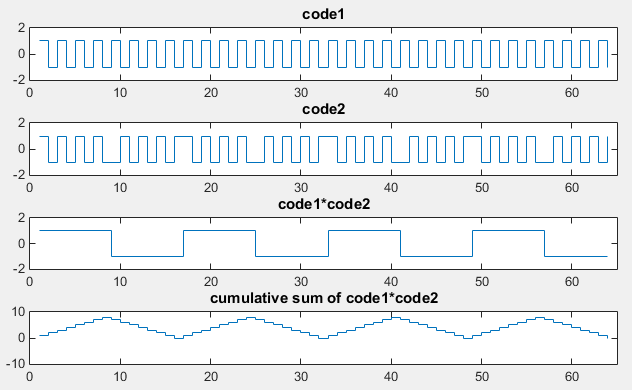Communication - Walsh Code                                 Home : www.sharetechnote.com Walsh Code   Walsh Code is a special set of code that is usually used for spreading digital data. One of the key characteristics of Walsh code is that all the members in the set is orthgonal to each other. One of the best known application of Walsh code is data spreading for CDMA and the code used in CDMA has 64 elements and each of the code is based on following table. Each Walsh code is based on each row of the table. If you check the orthogonality between rows in this table, you would see these rows are not orthogonal to each other. But if you replace 0 with '1' and '1' with '-1' (i.e, 0->1, 1->-1) or if you replace 0 with -1 and 1 with 1 (i.e, 0->-1, 1->1), you will see the resulting rows become orthogonal to each other.   < 3GPP2 C.S0002-0_v1.0 Table 2.1.3.1.8.1-1. 64-ary Orthogonal Symbol Set >How is it generated ?   Now let's see how this table is created. As is described in 3GPP2 C.S0002-0_v1.0 , these codes are created by an Hadamard matrix as shown below.With Matlab (or Octave), you can generate these table as shown below.   < Method 1 > H1 = 0; H2 = [H1 H1;H1 not(H1)]; H4 = [H2 H2;H2 not(H2)]; H8 = [H4 H4;H4 not(H4)]; H16 = [H8 H8;H8 not(H8)]; H32 = [H16 H16;H16 not(H16)]; H64 = [H32 H32;H32 not(H32)];   W64 = -1*(2 * H64-1); H64 would give you the table shown above in the form of matrix. W64 gives you the version of the table that has following mapping. 0 is replaced with 1 (i.e, 0 -> 1) 1 is replaced with -1 (i.e, 1 -> -1) Therefore, each row vector in W64 should be orthogonal to each other. You can easily check the orthogonality as follows. W64(1,:) * W64(2, :) will give you 0. It means row 1 and row 2 is orthogonal to each other. W64(1,:) * W64(3, :) will give you 0. It means row 1 and row 3 is orthogonal to each other. You can try any two rows and all of them should give you 0.     < Method 2 >   If you generate the matrix as shown below, you can produce W64 table directly (in stead of generating H matrix first and then mapping 0 -> 1, 1 -> -1). W1 = ; W2 = [W1 W1;W1 -W1]; W4 = kron(W2,W2); W8 = kron(W2,W4); W16 = kron(W2,W8); W32 = kron(W2,W16); W64 = kron(W2,W32); You can do orthogonality check for this matrix as well in following way. W64(1,:) * W64(2, :) will give you 0. It means row 1 and row 2 is orthogonal to each other. W64(1,:) * W64(3, :) will give you 0. It means row 1 and row 3 is orthogonal to each other. You can try any two rows and all of them should give you 0.   < Visualization of the code >   code1 = 2; code2 = 10; subplot(4,1,1); stairs(W64(code1,:));axis([0 65 -2 2]);title('code1'); subplot(4,1,2); stairs(W64(code2,:));axis([0 65 -2 2]);title('code2'); subplot(4,1,3); stairs(W64(code1,:) .* W64(code2,:));axis([0 65 -2 2]);title('code1*code2'); subplot(4,1,4); stairs(cumsum(W64(code1,:) .* W64(code2,:)));axis([0 65 -10 10]);title('cumulative sum of code1*code2');